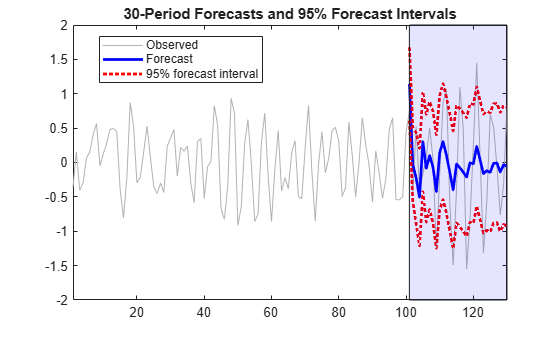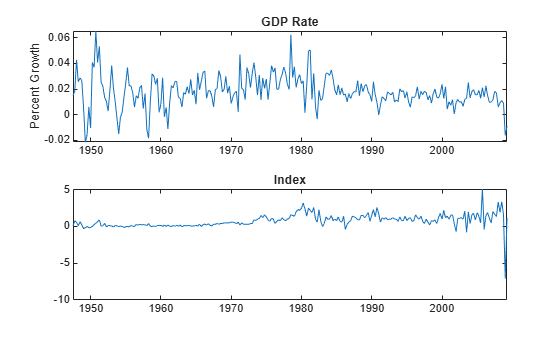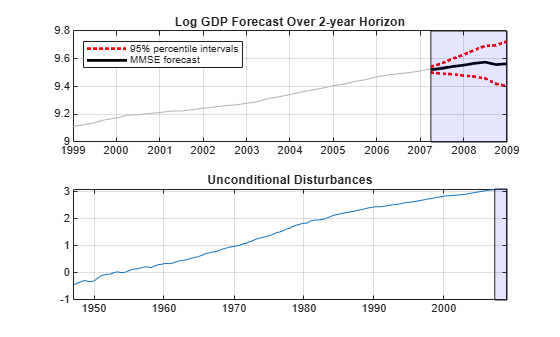Documentation

# forecast

Class: regARIMA

Forecast responses of regression model with ARIMA errors

## Syntax

[Y,YMSE] = forecast(Mdl,numperiods) [Y,YMSE,U] = forecast(Mdl,numperiods) [Y,YMSE,U] = forecast(Mdl,numperiods,Name,Value) 

## Description

[Y,YMSE] = forecast(Mdl,numperiods) forecasts responses (Y) for a regression model with ARIMA time series errors and generates corresponding mean square errors (YMSE).

[Y,YMSE,U] = forecast(Mdl,numperiods) additionally forecasts unconditional disturbances for a regression model with ARIMA errors.

[Y,YMSE,U] = forecast(Mdl,numperiods,Name,Value) forecasts with additional options specified by one or more Name,Value pair arguments.

## Input Arguments

expand all

Regression model with ARIMA errors, specified as a regARIMA model returned by regARIMA or estimate.

The properties of Mdl cannot contain NaNs.

Forecast horizon, or the number of time points in the forecast period, specified as a positive integer.

Data Types: double

### Name-Value Pair Arguments

Specify optional comma-separated pairs of Name,Value arguments. Name is the argument name and Value is the corresponding value. Name must appear inside quotes. You can specify several name and value pair arguments in any order as Name1,Value1,...,NameN,ValueN.

Presample innovations that initialize the moving average (MA) component of the ARIMA error model, specified as the comma-separated pair consisting of 'E0' and a numeric column vector or numeric matrix. forecast assumes that the presample innovations have a mean of 0.

• If E0 is a column vector, then forecast applies it to each forecasted path.

• If E0, Y0, and U0 are matrices with multiple paths, then they must have the same number of columns.

• E0 requires at least Mdl.Q rows. If E0 contains extra rows, then forecast uses the latest presample innovations. The last row contains the latest presample innovation.

By default, if U0 contains at least Mdl.P + Mdl.Q rows, then forecast infers E0 from U0. If U0 has an insufficient number of rows, and forecast cannot infer sufficient observations of U0 from the presample data (Y0 and X0), then E0 is 0.

Data Types: double

Presample unconditional disturbances that initialize the autoregressive (AR) component of the ARIMA error model, specified as the comma-separated pair consisting of 'U0' and a numeric column vector or numeric matrix. If you do not specify presample innovations E0, forecast uses U0 to infer them.

• If U0 is a column vector, then forecast applies it to each forecasted path.

• If U0, Y0, and E0 are matrices with multiple paths, then they must have the same number of columns.

• U0 requires at least Mdl.P rows. If U0 contains extra rows, then forecast uses the latest presample unconditional disturbances. The last row contains the latest presample unconditional disturbance.

By default, if the presample data (Y0 and X0) contains at least Mdl.P rows, then forecast infers U0 from the presample data. If you do not specify presample data, then all required presample unconditional disturbances are 0.

Data Types: double

Presample predictor data that initializes the model for forecasting, specified as the comma-separated pair consisting of 'X0' and a numeric matrix. The columns of X0 are separate time series variables. forecast uses X0 to infer presample unconditional disturbances U0. Therefore, if you specify U0, forecast ignores X0.

• If you do not specify U0, then X0 requires at least Mdl.P rows to infer U0. If X0 contains extra rows, then forecast uses the latest observations. The last row contains the latest observation of each series.

• X0 requires the same number of columns as the length of Mdl.Beta.

• If you specify X0, then you must also specify XF.

• forecast treats X0 as a fixed (nonstochastic) matrix.

Data Types: double

Forecasted or future predictor data, specified as the comma-separated pair consisting of 'XF' and a numeric matrix.

The columns of XF are separate time series, each corresponding to forecasts of the series in X0. Row t of XF contains the t-period-ahead forecasts of X0.

If you specify X0, then you must also specify XF. XF and X0 require the same number of columns. XF must have at least numperiods rows. If XF exceeds numperiods rows, then forecast uses the first numperiods forecasts.

forecast treats XF as a fixed (nonstochastic) matrix.

By default, forecast does not include a regression component in the model, regardless of the presence of regression coefficients in Mdl.

Data Types: double

Presample response data that initializes the model for forecasting, specified as the comma-separated pair consisting of 'Y0' and a numeric column vector or numeric matrix. forecast uses Y0 to infer presample unconditional disturbances U0. Therefore, if you specify U0, forecast ignores Y0.

• If Y0 is a column vector, forecast applies it to each forecasted path.

• If Y0, E0, and U0 are matrices with multiple paths, then they must have the same number of columns.

• If you do not specify U0, then Y0 requires at least Mdl.P rows to infer U0. If Y0 contains extra rows, then forecast uses the latest observations. The last row contains the latest observation.

Data Types: double

### Notes

• NaNs in E0, U0, X0, XF, and Y0 indicate missing values and forecast removes them. The software merges the presample data sets (E0, U0, X0, and Y0), then uses list-wise deletion to remove any NaNs. forecast similarly removes NaNs from XF. Removing NaNs in the data reduces the sample size. Such removal can also create irregular time series.

• forecast assumes that you synchronize presample data such that the latest observation of each presample series occurs simultaneously.

• Set X0 to the same predictor matrix as X used in the estimation, simulation, or inference of Mdl. This assignment ensures correct inference of the unconditional disturbances, U0.

• To include a regression component in the response forecast, you must specify the forecasted predictor data XF. That is, you can specify XF without also specifying X0, but forecast issues an error when you specify X0 without also specifying XF.

## Output Arguments

expand all

Minimum mean square error (MMSE) forecasts of the response data, returned as a numeric matrix. Y has numperiods rows and numPaths columns.

• If you do not specify Y0, E0, and U0, then Y is a numperiods column vector.

• If you specify Y0, E0, and U0, all having numPaths columns, then Y is a numperiods-by-numPaths matrix.

• Row i of Y contains the forecasts for the ith period.

Data Types: double

Mean square errors (MSEs) of the forecasted responses, returned as a numeric matrix. YMSE has numperiods rows and numPaths columns.

• If you do not specify Y0, E0, and U0, then YMSE is a numperiods column vector.

• If you specify Y0, E0, and U0, all having numPaths columns, then YMSE is a numperiods-by-numPaths matrix.

• Row i of YMSE contains the forecast error variances for the ith period.

• The predictor data does not contribute variability to YMSE because forecast treats XF as a nonstochastic matrix.

• The square roots of YMSE are the standard errors of the forecasts of Y.

Data Types: double

Minimum mean square error (MMSE) forecasts of future ARIMA error model unconditional disturbances, returned as a numeric matrix. U has numperiods rows and numPaths columns.

• If you do not specify Y0, E0, and U0, then U is a numperiods column vector.

• If you specify Y0, E0, and U0, all having numPaths columns, then U is a numperiods-by-numPaths matrix.

• Row i of U contains the forecasted unconditional disturbances for the ith period.

Data Types: double

## Examples

expand all

Forecast responses from the following regression model with ARMA(2,1) errors over a 30-period horizon:

$\begin{array}{l}\begin{array}{c}{y}_{t}={X}_{t}\left[\begin{array}{c}0.1\\ -0.2\end{array}\right]+{u}_{t}\\ {u}_{t}=0.5{u}_{t-1}-0.8{u}_{t-2}+{\epsilon }_{t}-0.5{\epsilon }_{t-1},\end{array}\end{array}$

where ${\epsilon }_{t}$ is Gaussian with variance 0.1.

Specify the model. Simulate responses from the model and two predictor series.

Mdl = regARIMA('Intercept',0,'AR',{0.5 -0.8},... 'MA',-0.5,'Beta',[0.1 -0.2],'Variance',0.1); rng(1); % For reproducibility X = randn(130,2); y = simulate(Mdl,130,'X',X);

Fit the model to the first 100 observations, and reserve the remaining 30 observations to evaluate forecast performance.

ToEstMdl = regARIMA('ARLags',1:2); EstMdl = estimate(ToEstMdl,y(1:100),'X',X(1:100,:));
 Regression with ARMA(2,0) Error Model (Gaussian Distribution): Value StandardError TStatistic PValue ________ _____________ __________ __________ Intercept 0.004358 0.021314 0.20446 0.83799 AR{1} 0.36833 0.067103 5.4891 4.0408e-08 AR{2} -0.75063 0.090865 -8.2609 1.4453e-16 Beta(1) 0.076398 0.023008 3.3205 0.00089863 Beta(2) -0.1396 0.023298 -5.9919 2.0741e-09 Variance 0.079876 0.01342 5.9522 2.6453e-09 
[yF,yMSE] = forecast(EstMdl,30,'Y0',y(1:100),... 'X0',X(1:100,:),'XF',X(101:end,:));

EstMdl is a new regARIMA model containing the estimates. The estimates are close to their true values.

Use EstMdl to forecast a 30-period horizon. Visually compare the forecasts to the holdout data using a plot.

[yF,yMSE] = forecast(EstMdl,30,'Y0',y(1:100),... 'X0',X(1:100,:),'XF',X(101:end,:)); figure plot(y,'Color',[.7,.7,.7]); hold on plot(101:130,yF,'b','LineWidth',2); plot(101:130,yF+1.96*sqrt(yMSE),'r:',... 'LineWidth',2); plot(101:130,yF-1.96*sqrt(yMSE),'r:','LineWidth',2); h = gca; ph = patch([repmat(101,1,2) repmat(130,1,2)],... [h.YLim fliplr(h.YLim)],... [0 0 0 0],'b'); ph.FaceAlpha = 0.1; legend('Observed','Forecast',... '95% Forecast Interval','Location','Best'); title(['30-Period Forecasts and Approximate 95% '... 'Forecast Intervals']) axis tight hold offMany observations in the holdout sample fall beyond the 95% forecast intervals. Two reasons for this are:

• The predictors are randomly generated in this example. estimate treats the predictors as fixed. Subsequently, the 95% forecast intervals based on the estimates from estimate do not account for the variability in the predictors.

• By shear chance, the estimation period seems less volatile than the forecast period. estimate uses the less volatile estimation period data to estimate the parameters. Therefore, forecast intervals based on the estimates should not cover observations that have an underlying innovations process with larger variability.

Forecast stationary, log GDP using a regression model with ARMA(1,1) errors, including CPI as a predictor.

Load the U.S. macroeconomic data set and preprocess the data.

load Data_USEconModel; logGDP = log(DataTable.GDP); dlogGDP = diff(logGDP); % For stationarity dCPI = diff(DataTable.CPIAUCSL); % For stationarity numObs = length(dlogGDP); gdp = dlogGDP(1:end-15); % Estimation sample cpi = dCPI(1:end-15); T = length(gdp); % Effective sample size frstHzn = T+1:numObs; % Forecast horizon hoCPI = dCPI(frstHzn); % Holdout sample dts = dates(2:end); % Date nummbers

Fit a regression model with ARMA(1,1) errors.

ToEstMdl = regARIMA('ARLags',1,'MALags',1); EstMdl = estimate(ToEstMdl,gdp,'X',cpi);
 Regression with ARMA(1,1) Error Model (Gaussian Distribution): Value StandardError TStatistic PValue __________ _____________ __________ __________ Intercept 0.014793 0.0016289 9.0818 1.0684e-19 AR{1} 0.57601 0.10009 5.7548 8.6754e-09 MA{1} -0.15258 0.11978 -1.2738 0.20272 Beta(1) 0.0028972 0.0013989 2.071 0.038355 Variance 9.5734e-05 6.5562e-06 14.602 2.723e-48 

Forecast the GDP rate over a 15-quarter horizon. Use the estimation sample as a presample for the forecast.

[gdpF,gdpMSE] = forecast(EstMdl,15,'Y0',gdp,... 'X0',cpi,'XF',hoCPI);

Plot the forecasts and 95% forecast intervals.

figure h1 = plot(dts(end-65:end),dlogGDP(end-65:end),... 'Color',[.7,.7,.7]); datetick hold on h2 = plot(dts(frstHzn),gdpF,'b','LineWidth',2); h3 = plot(dts(frstHzn),gdpF+1.96*sqrt(gdpMSE),'r:',... 'LineWidth',2); plot(dts(frstHzn),gdpF-1.96*sqrt(gdpMSE),'r:','LineWidth',2); ha = gca; title(['{\bf Forecasts and Approximate 95% }'... '{\bf Forecast Intervals for GDP rate}']); ph = patch([repmat(dts(frstHzn(1)),1,2) repmat(dts(frstHzn(end)),1,2)],... [ha.YLim fliplr(ha.YLim)],... [0 0 0 0],'b'); ph.FaceAlpha = 0.1; legend([h1 h2 h3],{'Observed GDP rate','Forecasted GDP rate ',... '95% Forecast Interval'},'Location','Best','AutoUpdate','off'); axis tight hold offForecast unit root nonstationary, log GDP using a regression model with ARIMA(1,1,1) errors, including CPI as a predictor and a known intercept.

Load the U.S. Macroeconomic data set and preprocess the data.

load Data_USEconModel; numObs = length(DataTable.GDP); logGDP = log(DataTable.GDP(1:end-15)); cpi = DataTable.CPIAUCSL(1:end-15); T = length(logGDP); % Effective sample size frstHzn = T+1:numObs; % Forecast horizon hoCPI = DataTable.CPIAUCSL(frstHzn); % Holdout sample

Specify the model for the estimation period.

ToEstMdl = regARIMA('ARLags',1,'MALags',1,'D',1);

The intercept is not identifiable in a model with integrated errors, so fix its value before estimation. One way to do this is to estimate the intercept using simple linear regression.

Reg4Int = [ones(T,1), cpi]\logGDP; intercept = Reg4Int(1);

Consider performing a sensitivity analysis by using a grid of intercepts.

Set the intercept and fit the regression model with ARIMA(1,1,1) errors.

ToEstMdl.Intercept = intercept; EstMdl = estimate(ToEstMdl,logGDP,'X',cpi,... 'Display','off')
EstMdl = regARIMA with properties: Description: "ARIMA(1,1,1) Error Model (Gaussian Distribution)" Distribution: Name = "Gaussian" Intercept: 5.80142 Beta: [0.00396701] P: 2 D: 1 Q: 1 AR: {0.922709} at lag  SAR: {} MA: {-0.387844} at lag  SMA: {} Variance: 0.000108943 Regression with ARIMA(1,1,1) Error Model (Gaussian Distribution) 

Forecast GDP over a 15-quarter horizon. Use the estimation sample as a presample for the forecast.

[gdpF,gdpMSE] = forecast(EstMdl,15,'Y0',logGDP,... 'X0',cpi,'XF',hoCPI);

Plot the forecasts and 95% forecast intervals.

figure h1 = plot(dates(end-65:end),log(DataTable.GDP(end-65:end)),... 'Color',[.7,.7,.7]); datetick hold on h2 = plot(dates(frstHzn),gdpF,'b','LineWidth',2); h3 = plot(dates(frstHzn),gdpF+1.96*sqrt(gdpMSE),'r:',... 'LineWidth',2); plot(dates(frstHzn),gdpF-1.96*sqrt(gdpMSE),'r:',... 'LineWidth',2); ha = gca; title(['{\bf Forecasts and Approximate 95% }'... '{\bf Forecast Intervals for log GDP}']); ph = patch([repmat(dates(frstHzn(1)),1,2) repmat(dates(frstHzn(end)),1,2)],... [ha.YLim fliplr(ha.YLim)],... [0 0 0 0],'b'); ph.FaceAlpha = 0.1; legend([h1 h2 h3],{'Observed GDP','Forecasted GDP',... '95% Forecast Interval'},'Location','Best','AutoUpdate','off'); axis tight hold offThe unconditional disturbances, ${u}_{t}$, are nonstationary, therefore the widths of the forecast intervals grow with time.

expand all

## Algorithms

• forecast computes the forecasted response MSEs, YMSE, by treating the predictor data matrices (X0 and XF) as nonstochastic and statistically independent of the model innovations. Therefore, YMSE reflects the variance associated with the unconditional disturbances of the ARIMA error model alone.

• forecast uses Y0 and X0 to infer U0. Therefore, if you specify U0, forecast ignores Y0 and X0.

## References

 Box, G. E. P., G. M. Jenkins, and G. C. Reinsel. Time Series Analysis: Forecasting and Control. 3rd ed. Englewood Cliffs, NJ: Prentice Hall, 1994.

 Davidson, R., and J. G. MacKinnon. Econometric Theory and Methods. Oxford, UK: Oxford University Press, 2004.

 Enders, W. Applied Econometric Time Series. Hoboken, NJ: John Wiley & Sons, Inc., 1995.

 Hamilton, J. D. Time Series Analysis. Princeton, NJ: Princeton University Press, 1994.

 Pankratz, A. Forecasting with Dynamic Regression Models. John Wiley & Sons, Inc., 1991.

 Tsay, R. S. Analysis of Financial Time Series. 2nd ed. Hoboken, NJ: John Wiley & Sons, Inc., 2005.

Download ebook